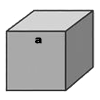Home|Add to Favorites|Add to My Toolkit Engineering MathVolume Calculator|Register|Sign in|CustomizationCalculate Cube Volume(Cube Volume Calcularor)

A cube is a rectangular solid with 6 square faces and all edges are equal. To calculate cubic volume,  please enter a numeric  length below, the answer is returned as a number of cubic units.
Custom Search
 Cube Volume Calculator#nav{position:fixed; top:20px; left:30px;width:86px;height: 67px; border:1px solid #aaa;display: none;background: #fafafa;padding-left: 8px;padding-right: 8px;padding-top: 12px}Length  Volume The formula for the volume of a cube: a3     a = length of any side (or edge) Top Use:Calculate Cube Volume - Length:3.125Recent user inquiry:2022/1/28 03:06Calculate Cube Volume - Length:122022/1/27 22:27Calculate Cube Volume - Length:11002022/1/27 18:06Calculate Cube Volume - Length:182022/1/27 10:48Calculate Cube Volume - Length:12.002022/1/27 09:41Calculate Cube Volume - Length:45